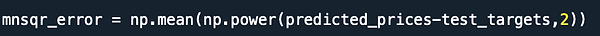top of page

## Feed Forward Neural Network

It was already described what a Feed Forward Neural Network was. Below we will display how to program a Feed Forward Neural Network. The steps are similar to a convolutional neural network with a few adjustments.

1. Import all needed data

\

\2. Import the data and set a percentage of the data equal to train and then to test to compute the optimized weights and accuracy. The data is set to understandable variable names, just for preference

3. Create the model and add any amount of layers, in this example there is one hidden layer

4. Train the model on the train data, like in logistic regression, and set any needed amount of iterations (epochs). Batch size is the amount of training examples used in each iteration.

5. ​Set the loss metric (mean squared error) and predict the model

6. Find the mean squared error

​  7. Plot the cost per iterations to find the accuracy of the model

bottom of page